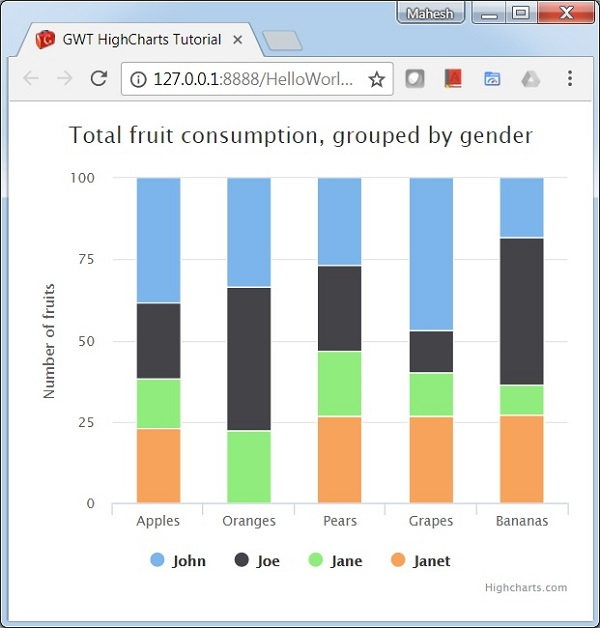# Column with stacked percentage

Following is an example of a stacked column Chart with percentages.

We have already seen the configuration used to draw a chart in Highcharts Configuration Syntax chapter. Let us now see additional configurations and also how we have added stacking attribute in plotoptions.

An example of a stacked column Chart with percentages is given below.

## plotOptions

The plotOptions is a wrapper object for configurations objects for each series type. The configuration objects for each series can also be overridden for each series item as given in the series array. This is to stack the values of each series on top of each other. This is to stack the values of each series on top of each other.

Configure the stacking of the chart using plotOptions.column.stacking as "percent". Possible values are null which disables stacking, "normal" stacks by value and "percent" stacks the chart by percentages.

```chart.setColumnPlotOptions(new ColumnPlotOptions()
.setStacking(Stacking.PERCENT)
);
```

## Example

HelloWorld.java

```package com.tutorialspoint.client;

import org.moxieapps.gwt.highcharts.client.Chart;
import org.moxieapps.gwt.highcharts.client.Series.Type;
import org.moxieapps.gwt.highcharts.client.ToolTip;
import org.moxieapps.gwt.highcharts.client.ToolTipData;
import org.moxieapps.gwt.highcharts.client.ToolTipFormatter;
import org.moxieapps.gwt.highcharts.client.plotOptions.ColumnPlotOptions;
import org.moxieapps.gwt.highcharts.client.plotOptions.PlotOptions.Stacking;

public class HelloWorld implements EntryPoint {
final Chart chart = new Chart()
.setType(Type.COLUMN)
.setChartTitleText("Total fruit consumption, grouped by gender")
.setColumnPlotOptions(new ColumnPlotOptions()
.setStacking(Stacking.PERCENT)
)
.setToolTip(new ToolTip()
.setFormatter(new ToolTipFormatter() {
@Override
public String format(ToolTipData toolTipData) {
" ("+ Math.round(toolTipData.getPercentage()) + "%)";
}
})
);

chart.getXAxis()
.setCategories("Apples", "Oranges", "Pears", "Grapes", "Bananas");

chart.getYAxis()
.setAllowDecimals(false)
.setMin(0)
.setAxisTitleText("Number of fruits");

.setName("John")
.setPoints(new Number[] {5, 3, 4, 7, 2})
);
.setName("Joe")
.setPoints(new Number[] {3, 4, 4, 2, 5})
);
.setName("Jane")
.setPoints(new Number[] {2, 2, 3, 2, 1})
);
.setName("Janet")
.setPoints(new Number[] {3, 0, 4, 4, 3})
);